Students can Download Tamil Nadu 12th Chemistry Model Question Paper 3 English Medium Pdf, Tamil Nadu 12th Chemistry Model Question Papers helps you to revise the complete Tamilnadu State Board New Syllabus, helps students complete homework assignments and to score high marks in board exams.

## TN State Board 12th Chemistry Model Question Paper 3 English Medium

Time: 3 Hours
Maximum Marks: 70

Instructions:

1. The question paper comprises of four parts
2. You are to attempt all the parts An internal choice of questions is provided wherever applicable
3. All questions of Part I, II, III, and IV are to be attempted separately
4. Question numbers 1 to 15 in Part I are Multiple Choice Questions of one mark each. These are to be answered by choosing the most suitable answer from the given four alternatives and writing the option code and the corresponding answer
5. Question numbers 16 to 24 in Part II are two-mark questions. These are lo be answered in about one or two sentences
6. Question numbers 25 to 33 in Part III are three-marks questions. These are to be answered in about three to five short sentences
7. Question numbers 34 to 38 in Part IV are five-mark Questions These are to answered in detail. Draw diagrams wherever necessary

Part – 1

Answer all the questions. Choose the correct answer. [15 × 1 = 15]

Question 1.
Wolframite ore is separated from tinstone by the process of
(a) Smelting
(b) Calcination
(c) Roasting
(d) Electromagnetic separation
(d) Electromagnetic separation

Question 2.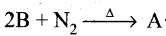Identify A
(a) BN3
(b) B3N
(c) (BN)3
(d) BN
(d) BN

Question 3.
Among the following the correct order of acidity is
(a) HClO2 < HCIO < HClO3 < HClO4
(b) HClO4 < HClO2 < HClO < HClO3
(c) HClO3 < HClO4 < HClO2 < HClO
(d) HClO < HClO2 < HClO3 < HClO4
(d) HClO < HClO2 < HClO3 < HClO4

Question 4.
Which of the following transition metal is present in Vitamin B12 ?
(a) Cobalt
(b) Platinum
(c) Copper
(d) Iron
(a) CobaltQuestion 5.
A magnetic moment of 1.73BM will be shown by one among the following.
(a)TiCl4
(b) [COCl6]4-
(c) [CU(NH3)4]2+
(d) [Ni(CN)4]2-
(c) [CU(NH3)4]2+

Question 6.
Consider the following statements.
(,i) metallic solids possess high electrical and thermal conductivity
(ii) solid ice are soft solids under room temperature
(iii) In non polar molecular solids constituent molecules are held together by strong electrostatic forces of attraction
Which of the above statements is./ are not correct?
(a) (i) & (ii)only
(b) (iii) only
(c) (ii) only
(d) (i) only
(b) (iii) only

Question 7.
For a first order reaction, the rate constant is 6.909 min-1.The time taken for 75% conversion
in minutes is …………………………..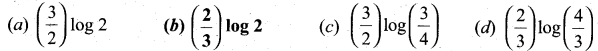(b) $$\left(\frac{2}{3}\right) \log 2$$
Solution: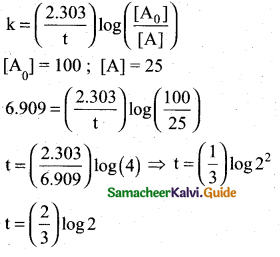Question 8.
The pH of 10-5 M KOH solution will be ……………….
(a) 9
(b) 5
(c) 19
(d) none of these
(a) 9

Solution:
KOH → K+ + OH
10-5M 10-5M 10-5M
[OH]= 10-5M
pH = 14 – pOH
pH = 14 – (-log [OH])
= 14 + log [OH ] = 14 + log10-5
= 14 – 5 = 9

Question 9.
The Lead storage battery is used in
(a) pacemakers
(b) automobiles
(c) electronic watches
(d) flash light
(b) automobiles

Question 10.
The coagulation values in millimoles per litre of the electrolytes used for the coagulation of AS2S3 are given below
(I) (NaCl) = 52
(II) (BaCl) = 0.69
(III) (MgSO4) = 0.22
The correct order of their coagulating power is
(a) III > II > I
(b) I > II > III
(c) I > III > II
(d) II > III> I
Hint: coagulating power ± $$\frac{1}{\text { coagulation value }}$$
(a) III > II > I

Question 11.
Oxygen atom in ether is
(a) very active
(b) replacable
(c) comparatively inert
(d) less active
(c) comparatively inert

Question 12.
During nucleophilic addition reaction, the hybridisation of carbon changes from
(a) sp2 to sp3
(b) sp3 to sp2
(c) sp to sp3
(d) dsp2 to sp3
(a) sp2 to sp3

Question 13.
Match the following: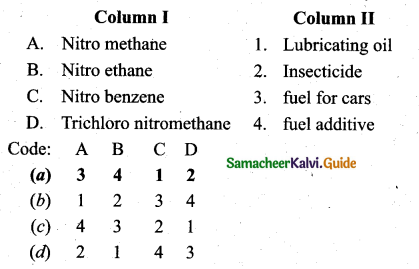(a) A – 3, B – 4, C – 1, D – 2

Question 14.
Which one of the following is levorotatory?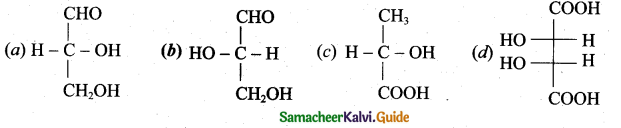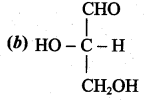Question 15.
Which one of the following structures represents nylon 6,6 polymer?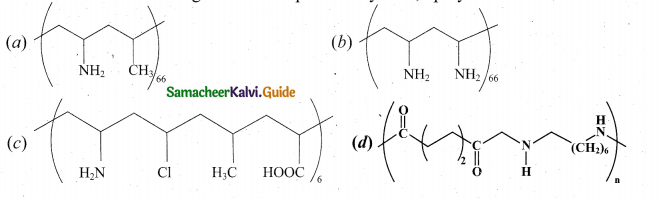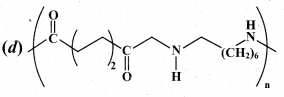Part – II

Answer any six questions. Question No. 23 is compulsory. [6 × 2 = 12]

Question 16.
Carbon monoxide is more effective reducing agent than carbon below 983 K but, above this temperature, the reverse is true -Explain.
From the Ellingham diagram, we find that at 983 K, the curves intersect.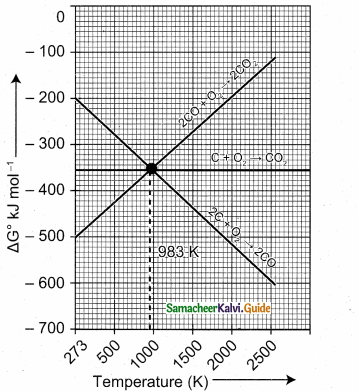The value of ∆G° for change of C to CO2 is less than the value of ∆G° for change of CO to CO2 Therefore, coke (C) is a better reducing agent than CO at 983K or above temperature. However below this temperature (e.g. at 673K), CO is more effective reducing agent than C.

Question 17.
Mention the uses of the potash alum.

• It is used for purification of water
• It is also used for water proofing and textiles
• It is used in dyeing, paper and leather tanning industries
• It is employed as a styptic agent to arrest bleeding.

Question 18.
Why Gd3+ is colourless?
Gd – Electronic Configuration : [Xe] 4f75d1 6s2
Gd3+ Electronic Configuration : [Xe] 4f7
In Gd3+ , no electrons are there in outer d-orbitals. d-d transition is not possible. So it is colourless.Question 19.
Ionic solids conduct electricity in molten state but not in solid state. Explain.
In the molten state, ionic solids dissociate to give free ions and hence can conduct electricity. However in the solid state, as the ions are not free to move but remain held together by strong electrostatic forces of attraction, so they cannot conduct electricity in the solid state.

Question 20.
What are Lewis acids and bases? Give two example for each.
I. Lewis acids:
(i) Lewis acid is a species that accepts an electron pair.
(ii) Lewis acid is a positive ion (or) an electron deficient molecule.
(iii) Example, Fe2+, CO2, BF3 , SiF4 etc…

II. Lewis bases:
(i) Lewis base is a species that donates an electron pair.
(ii) Lewis base is an anion (or) neutral molecule with atleast one lone pair of electrons.
(iii) Example, NH3, F, CH2= CH2 CaO etc….

Question 21.

• The nature of adsorbate can influence the adsorption. Gases like NH, C02 are easily liquefiable as have greater Van der Waals forces of attraction and hence readily adsorbed due to high critical temperature.
• But permanent gases like H2. N2 can not be easily liquefied and having low critical temperature and adsorbed slowly.

Question 22.
Alcohol can act as Bronsted base. Prove this statement.
Alcohols can also act as a Bronsted bases. It is due to the presence of unshared electron pairs on oxygen which make them to accept proton. So proton acceptor are Bronsted bases. i. e., alcohols are Bronsted bases.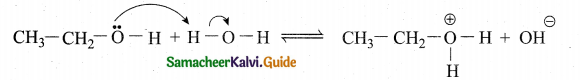Question 23. Arrange the following in decreasing order of basic strength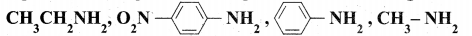(i) Aliphatic amines are more basic than aromatic amines. Therefore CH3CH2NH2 and CH3NH2 are more basic. Among the ethylamine and methylamine, ethylamine was experienced more +1 effect than methylamine and hence ethylamine is more basic than methylamine.

(ii) Nitrogroup has a powerfol electron withdrawing group and they have both -R effect as well as -I effect. As a result, all the nitro anilines are weaker bases than aniline. In P-nitroaniline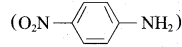both R effect and -I effect of the NO2 group decrease the basicity.

(iii) Therefore decreasing order of basic strength is,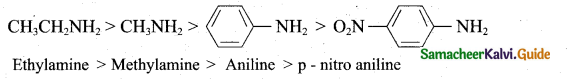Question 24.
How are biopolymers more beneficial than synthetic polymers?
Durability of synthetic polymers is advantageous, however it presents a serious waste disposable problem. In renewal of the disposable problem, biodegradable polymers are useful to us.

Biopolymers are safe in use. They disintegrate by themselves in biological system during a certain period of time by enzymatic hydrolysis and to some extent by oxidation and hence, are biodegradable. As a result, they do not cause any pollution.

Part – III

Answer any six questions. Question No. 26 is compulsory. [6 × 3 = 18]

Question 25.
Explain Aluminothermic process.
Metallic oxides such as Cr2O3 can be reduced by an aluminothermic process. In this process, the metal oxide is mixed with aluminium powder and placed in a fire clay crucible. To initiate the reduction process, an ignition mixture (usually magnesium and barium peroxide) is used.

BaO2 + Mg → BaO + Mgo

During the above reaction a large amount of heat is evolved (temperature upto 2400°C, is generated and the reaction enthalpy is : 852 kJ-moF1) which facilitates the reduction of Cr2O3 by aluminium power.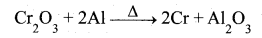Question 26.
Give a reason to support that sulphuric acid is a dehydrating agent.
A double salt which contains fourth period alkali metal (A) on heating at 500K gives (B). Aqueous solution of (B) gives white precipitate with BaCl2 and gives a red colour compound with alizarin. Identify A and B.
(i) A double salt which contains fourth period alkali metal (A) is potash alum
K2SO4 Al2 (SO4 )3 .24H2O

(ii) On heating potash alum (A) 500 K give anhydrous potash alum (or) burnt alum (B).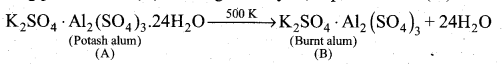(iii) Aqueous solution of burnt alum, has sulphates ion, potassium ion and aluminium ion.
Sulphate ion reacts with BaCl2 to form white precipitate of Barium Sulphate:
(SO4 )2- + BaCl2 → BaSO4 + 2Cl
Aluminium ion reacts with alizarin solution to give a red colour compound.

Question 27.
[Ti (H2O)6 ]2+ is purple in colour. Prove this statement.
(i) In [Ti (H2O)6 ]2+, the central metal ion is Ti3+ which has d1 configuration. This single electron occupies one of the ttgorbitals in the octahedral aqua ligand field.

(ii) When white light falls on this complex, the d electron absorbs light and promotes itself to eg level.

(iii) The spectral data show the absorption maximum is at 20000 cm-1 corresponding to the crystal field splitting energy (∆0) 239.7 kJ mol-1. The transmitted colour associated with this absorption is purple and hence the complex appears in purple in colour.Question 28.
Define half life of a reaction. Show that for a first order reaction half life is independent of initial concentration.
Half life of a reaction is defined as the time required for the reactant concentration to reach one half of its initial value.
For a first order reaction, the half life is a constant i.e., it does not depend on the initial concentration.
The rate constant for a first order reaction is given by,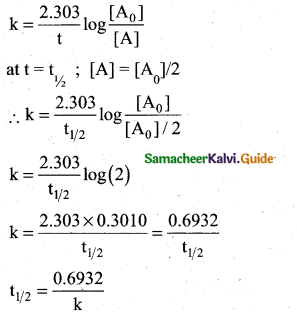Question 29.
State Kohlrausch Law. How is it useful to determine the molar conductivity of weak electrolyte at infinite dilution.
Kohlrausch’s law: It is defined as, at infinite dilution the limiting molar conductivity of an electrolyte is equal to the sum of the limiting molar conductivities of its constituent ions.

Determination of the molar conductivity of weak electrolyte at infinite dilution:
It is impossible to determine the molar conductance at infinite dilution for weak electrolytes experimentally. However, the same can be calculated using Kohlraush’s Law. For example, the molar conductance of CH3COOH, can be calculated using the experimentally determined molar conductivities of strong electrolytes HCl, NaCl and CH3COONa .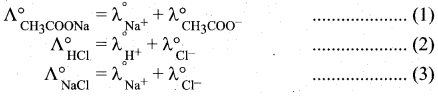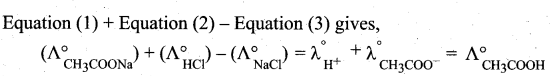Question 30.
Explain the following observations.
(a) Lyophilic colloid is more stable than lyophobic colloid.
(b) Coagulation takes place when sodium chloride solution added to a colloidal solution of ferric hydroxide.
(c) Sky appears blue in colour.
(a) A lyophilic sol is stable due to the charge and the hydration of the sol particles. Such a sol can only be coagulatd by removing the water and adding solvents like alcohol, acetone, etc. and then an electrolyte. On the other hand, a lyophobic sol is stable due to charge only and hence it can be easily coagulated by adding small amount of an electrolyte.

(b) The colloidal particles get precipitated, i.e., ferric hydroxide is precipitated.

(c) The atmospheric particles of colloidal range scatter blue component of the white sunlight preferentially. That is why the sky appears blue.

Question 31.
Mention the uses of formic acid?
Formic acid. It is used
(i) for the dehydration of hides.
(ii) as a coagulating agent for rubber latex
(iii) in medicine for treatment of gout
(iv) as an antiseptic in the preservation of fruit juice

Question 32.
Write the structure of all possible dipeptides which can be obtained from glycine and alanine.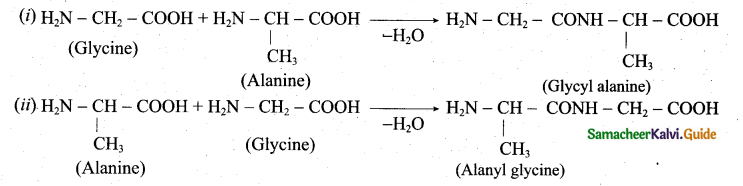Therefore two dipeptides structures are possible from glycine and alanine. They are (i) glycyl alanine and (ii) Alanyl glycine

Question 33.
How the tranquilizers work in body?

• They are neurologically active drugs.
• Tranquilizer acts on the central nervous system by blocking the neurotransitter dopamine in the brain.
• This drug is used for treatment of stress anxiety, depression, sleep disorders and severe mental diseases like schizophrenia.

Part – IV

Answer all the questions. [5 x 5 = 25]

Question 34.
(a) (i) How will you purify metals by using iodine?(3)
(ii) Boron does not react directly with hydrogen. Suggest one method to
prepare diborane from BF3(2)
[OR]
(b) (i) Write the reason for the anomalous behaviour of Nitrogen. (3)
(ii) Mn2+ is more stable than Mn4+. Why? (2)
(a) (i) This method is based on the thermal decomposition of metal compounds which lead to the formation of pure metals. Titanium and zirconium can be purified using this method. For example, the impure titanium metal is heated in an evacuated vessel with iodine at a temperature of 550 K to form the volatile titanium tetra-iodide.( TiI4 ). The impurities are left behind, as they do not react with iodine.
Ti(s) + 2I2(s) → TiI4 (vapour)

The volatile titanium tetraiodide vapour is passed over a tungsten filament at a
temperature around I 800 K. The titanium tetraiodide is decomposed and pure titanium
is deposited on the filament. The iodine is reused.
TiI4 (vapour) ) → Ti(s) +2I2(s)

(ii) Boron does not react directly with hydrogen. However it forms a variety of hydrides
called boranes. Treatment of gaseous boron triuluoride with sodium hydride around
450 K gives diborane.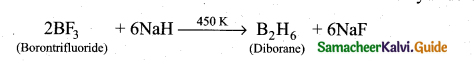(b) (i) 1. Due to its small size, high electro negativity, high ionisation enthalpy and absence of d-orbitals.

2. N2 has a unique ability to form pπ- pπ multiple bond whereas the heavier members of this group (15) do not form pπ- pπ bond, because their atomic orbitals are so large and diffused that they cannot have effective overlapping.

3. Nitrogen exists a diatomic molecule with triple bond between the two atoms whereas other elements form single bond in the elemental state.

4. N cannot form dπ- dπ bond due to the absence of d-orbitals whereas other elements can.

(ii) The relative stability of different oxidation states of 3d metals is correlated with the extra stability of half-filled and fully filled electronic configurations.
Example: Mn2+ (3d5) is more stable than Mn4+ (3d3)Question 35.
(a) (i) Draw all possible stereo isomers of a complex Ca[CO(NH3)Cl(Ox)2] (3)
(ii) What is Bragg’s equation? (2)
(b) (i) What is an elementary reaction? Give the differences between order and molecularity of a reaction. (3)
(ii) In a first order reaction A → products, 60% of the given sample of A decomposes in 40 min. what is the half life of the reaction? (2)
(a) (i) Possible stereo isomers of a complex Ca[CO(NH3)Cl(Ox)2]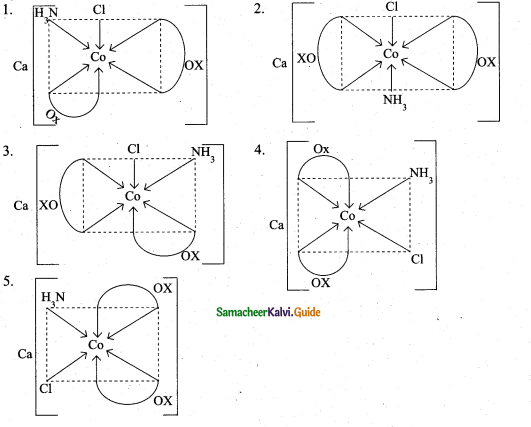(ii) 1. X-ray diffraction analysis is the most powerful tool for the determination of crystal structure.

2. The interplanar distance (d) between two successive planes of atoms can be calculated using the following equation form the X-ray diffraction data 2d sin θ = nλ. The equation is known as Bragg’s equation
Where λ = wavelength of X-ray; d = Interplanar distance
θ = The angle of diffraction n = order of reflection
By knowing the values of λ, λ and n, we can calculate the value of d
$$d=\frac{n \lambda}{2 \sin \theta}$$

Using these values, the edge of the unit cell can be calculated.

[OR]

(b) (i) Elementary reaction: Each and every single step in a reaction mechanism is called an elementary reaction.

Differences between order and molecularity:

Order of a reaction :

• It is the sum of the powers of concentration terms involved in the experimentally determined rate law.
• It can be zero (or) fractional (or) integer.
• It is assigned for a overall reaction.

Molecularity of a reaction :

• It is the total number of reactant species that are involved in an elementary step.
• It is always a whole number, cannot be zero or a fractional number.
• It is assigned for each elementary step of mechanism.

(ii)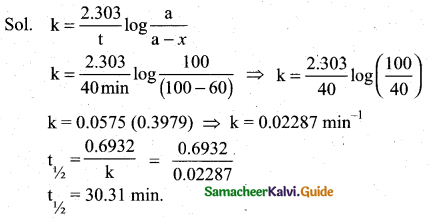Question 36.
(a) (i) Derive the relation between pH and pOH (3)
(ii) Give three uses of emulsions.(2)
[OR]
(b) How would you measure the conductivity of ionic solutions? (5)
(a) (i) pH = -log1o [H3O+] …………..(1)
pOH = -log1o [OH] ……..(2)
pH + pOH = (-log1o[H3O+]) + (-log1o [OH])
= -[(log10tHO+]) + (logio [OfT])]
pH + pOH = -log10[H3O+] [OH ]
[H3O+] [OH] = Kw
∴ pH + pOH = – log1oKw
pH + pOH = pKw [pkw = -log1oKw]

At 25°C, the ionic product of water Kw =1 x 10-14.
pKw = -log1o10-14 = 14log1o10= 14
pKw = 14
∴ pH + pOH = 14 at 25°C.

(ii)

1. The cleansing action of soap is due to emulsions.
2. It is used in the preparation of vanishing cream.
3. It is used in the preparation of cold liver oil.

[OR]

(b) The conductivity of an electrolytic solution is determined by using a wheatstone bridge arrangement in which one resistance is replaced by a conductivity cell filled with the electrolytic solution of unknown conductivity.

In the measurement of specific resistance of a metallic wire, a DC power supply is used. Here AC is used for this measurement to prevent electrolysis. Because DC current through the conductivity cell leads to the electrolysis of the solution taken in the cell.

A wheatstone bridge is constituted using known resistances P,Q, a variable resistance S and conductivity cell. An AC source (550 Hz to 5 KHz) is connected between the junctions A and C.
A suitable detector is connected between the junctions B and D.
The variable resistance S is adjusted until the bridge is balanced and in this conditions, there is no current flow through the detector.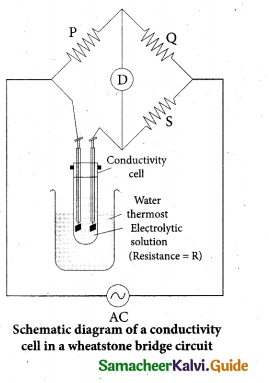Under balanced condition
P/Q = R/S
∴ R = P/Q x S …………(1)
The resistance of the electrolytic solution (R) is calculated from the known resistance values P, Q and the measured S value using the equation (1).

Specific conductance (or) conductivity of an electrolyte can be calculated from the resistance value (R) using the following expression
$$\kappa=\frac{1}{R}\left[\frac{l}{A}\right]$$

The value of cell constant $$\frac{l}{A}$$ is usually provided by the cell manufacturer. Alternatively the cell constant may be determined using KC1 solution whose concentration and specific conductance are known.Question 37.
(a) (1) What is metamerism? Give the structure and ¡UPAC name of metamers of 2-methoxy propane (2)
(ii) Explain the following reactions.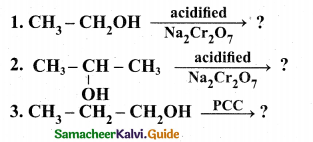(2)
(OR]
(b) An organic compound (A) of molecular formula C7H8O on oxidation with alkaline KMnO4 gives (B) of formula C7H6O.
(B) on reaction with Cl2 in the presence of catalyst FeCl3 gives
(C) of formula C7H5OCl. (B) on reaction with Cl in the absence
of catalyst gives C7H5OCl. Identify A,B,C,D and explain the reaction involved.
(a) (i) Metamerism: It is a special type of isomerism in which molecules with same formula, same functional group, but different only in the nature of the alkyl group attached to oxygen.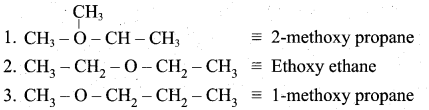Ethoxy ethane and 1-methoxy propane are metamers of 2-methoxy propane.

(ii)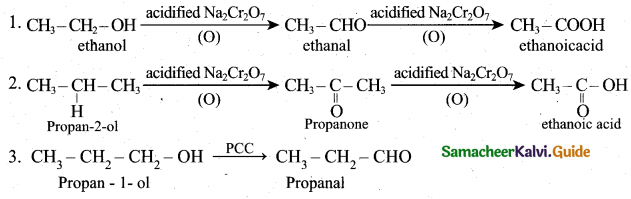(b)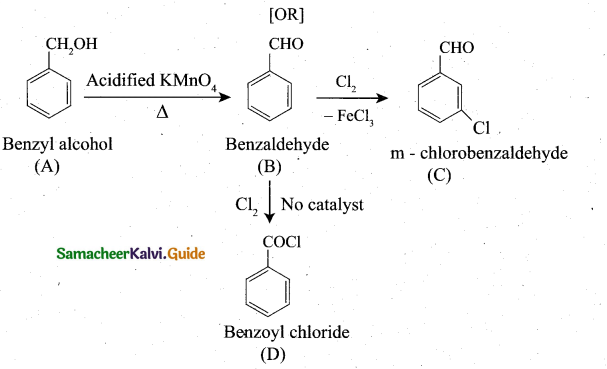Question 38.
(a) (0 Account for the following:
1. Primary amines (R – NH2)have higher boiling point than tertiary amines (R3N).
2. Aniline does not undergo Friedel-Crafts reaction.
3. (CH3)2NH is more basic than (CH3)3N in an aqueous solution. (3)
(H) Name two fat soluble vitamins, their sources and the diseases caused due to
their deficiency in diet. (2)
[OR]
(b) (i) Why ranitidine is a better antacid than magnesium hydroxide? (2)
(ii) What is bakelite? How is it prepared? Give its uses. (3)

(a) (i) 1. Due to maximum intermolecular hydrogen bonding in primary amines (due to presence of more number of H-atoms), primary amines have higher boiling point in comparison to tertiary amines.

2. Aniline does not undergo Friedel-Crafts reaction due to acid-base reaction. Aniline and a Lewis Acid / Protic Acid, which is used in Friedel-crafts reaction.

3. In (CH3)3N there is maximum steric hindrance and least solvation but in (CH3)2NH the solvation is more group; di-methyl amine is still a stronger base than trimethyl amine.

(ii)

 Vitamins Sources Deficiency Diseases Vitamin A Fish, liver oil, carrot Night blindness Vitamin D Sunlight, milk, egg yolk Rickets and osteomalacia

[OR]

(b) (i) To treat acidity, weak base such as magnesium hydroxide is used. But this weak base make the stomach alkaline and trigger the production of much acid. This treatment only relieves the symptoms and does not control the cause. But ranitine stimulate the secretion of HC1 by activating the receptor in the stomach wall which binds the receptor and inactivate them. So ranitine is a better antacid than magnesium hydroxide.

(ii) 1. Bakelite is a thermo setting plastic. It is prepared from the monomers such as phenol and formaldehyde. The condensation polymerisation take place in the presence of acid or base catalyst.

2. Phenol reacts with methanal to form ortho or para hydroxyl methyl phenols which on further reaction with phenol gives linear polymer called novolac. Novolac on further healing with formaldehyde undergoes cross linkages to form bakelite.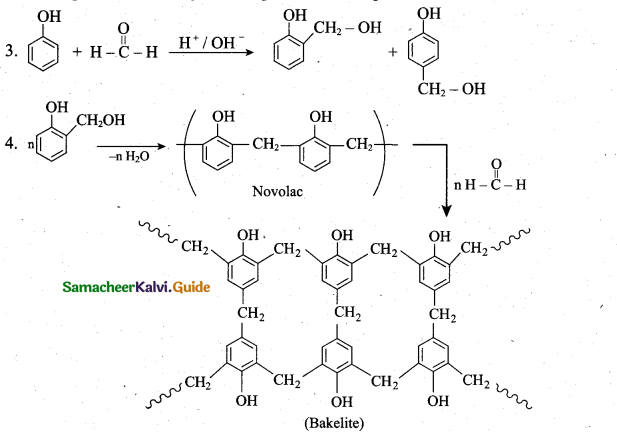• Novolac is used in paints ‘Soft bakelites are used in making glue for binding laminated
wooden planks and in varnishes
• Hard bakelites are used to prepare combs, pens.